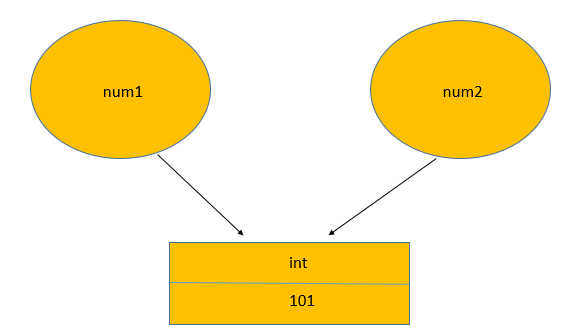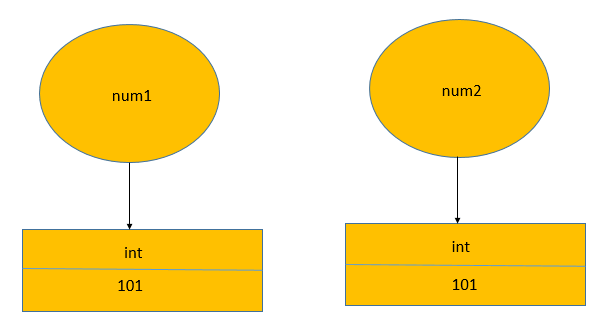Python Tutorial

# Python Identity Operators

Identity operators in Python serve the purpose of comparing the memory locations of two objects, particularly when both objects share the same name and can only be differentiated based on their memory location. The two identity operators, "is" and "is not," facilitate this comparison by determining if two variables refer to the same memory location or not. The "is" operator returns True if the variables refer to the same memory location and False otherwise, while the "is not" operator yields True if the variables point to different memory locations and False otherwise. These identity operators play a crucial role in efficiently managing and understanding object references in Python, contributing to more robust and optimized code.

1. is - Returns true if both variables are the same object.
2. is not - Returns true if both variables are not the same object.

In Python, the expression x is y is evaluated as True if and only if both x and y refer to the same object in memory. The id() function is used to determine the unique identifier (memory address) of an object, and it is employed by the "is" operator to compare the identities of two variables.## Python is operator

The "is" operator is used to check if two variables refer to the same object in memory. It evaluates to True if the variables have the same identity, meaning they are pointing to the same memory location, and False otherwise.

Syntax:
x is y

Where x and y are two variables or expressions.

x = '101' y = '101' z = '102' if (x is y): print ('x is y') else: print ('x is not y') if (x is z): print('x is z') else: print ('x is not z') if (y is z): print('y is z') else: print ('y is not z')
output
x is y x is not z y is not z

The "is" operator is particularly useful when you want to check if two variables reference the same object, and it is commonly used for object identity comparisons in Python. However, it is essential to use "is" judiciously, considering that object identity may not always imply equality, especially for mutable objects that may have the same content but are not the same object. For equality comparisons based on content, the "==" operator should be used.

## Python is not Operator

The "is not" operator is the negation of the "is" operator. It is used to check if two variables do not refer to the same object in memory. It evaluates to True if the variables have different identities, indicating they are pointing to different memory locations, and False otherwise.

Syntax:
x is not y

Where x and y are two variables or expressions.

x = '101' y = '101' z = '102' if (x is not y): print ('x is not y') else: print ('x not y') if (x is not z): print ('x is not z') else: print ('x is z') if (y is not z): print ('y is not z') else: print ('y is z')
output
x not y x is not z y is not z

The "is not" operator is valuable when you want to verify that two variables are not referring to the same object. It helps in distinguishing between separate objects and is often used for memory identity checks in Python. However, like the "is" operator, it is crucial to use "is not" appropriately, considering that different objects may have the same content but are not the same object. For content-based equality comparisons, the "!=" operator should be used.

## Is there a difference between "==" and "is"?

There is a fundamental difference between the "==" operator and the "is" operator in Python.

The "==" operator is used for content-based comparison, checking whether the values of two variables or expressions are the same. It evaluates to True if the values are equal, irrespective of whether they are the same object in memory or not. For example, a == b would return True if the values of variables 'a' and 'b' are the same.

On the other hand, the "is" operator is used for identity comparison, determining if two variables or expressions refer to the exact same object in memory. It evaluates to True only if the variables point to the same memory location, indicating they are the same object. For example, x is y would return True if 'x' and 'y' are the same object in memory.

The is operator will return True if two variables point to the same object, "==" if the objects referred to by the variables are equal: for example in the following case num1 and num2 refer to an int instance storing the value 101:

num1 = 101 num2 = num1Because num2 refers to the same object is and == will give True:

>>> num1 == num2 True >>> num1 is num2 True

In the following example the names num1 and num2 refer to different int instances, even if both store the same integer:

>>> num1 = 101 >>> num2 = 101Because the same value (integer) is stored == will return True, that's why it's often called value comparison . However is operator will return False because these are different objects :

>>> num1 == num2 True >>> num1 is num2 False

### You should only use is operator if you:

1. want to check if two objects are really the same object (not just the same "value").
1. want to compare a value to a Python constant.
The constants in Python are:
1. None
2. True
3. False
4. NotImplemented
5. Ellipsis
6. __debug__
7. classes (for example int is int or int is float)

### Conclusion

Python identity operators, "is" and "is not," are used to compare the memory locations of two variables or expressions. The "is" operator returns True if the variables refer to the same object in memory, while the "is not" operator returns True if the variables point to different memory locations, allowing for efficient object identity checks in Python.